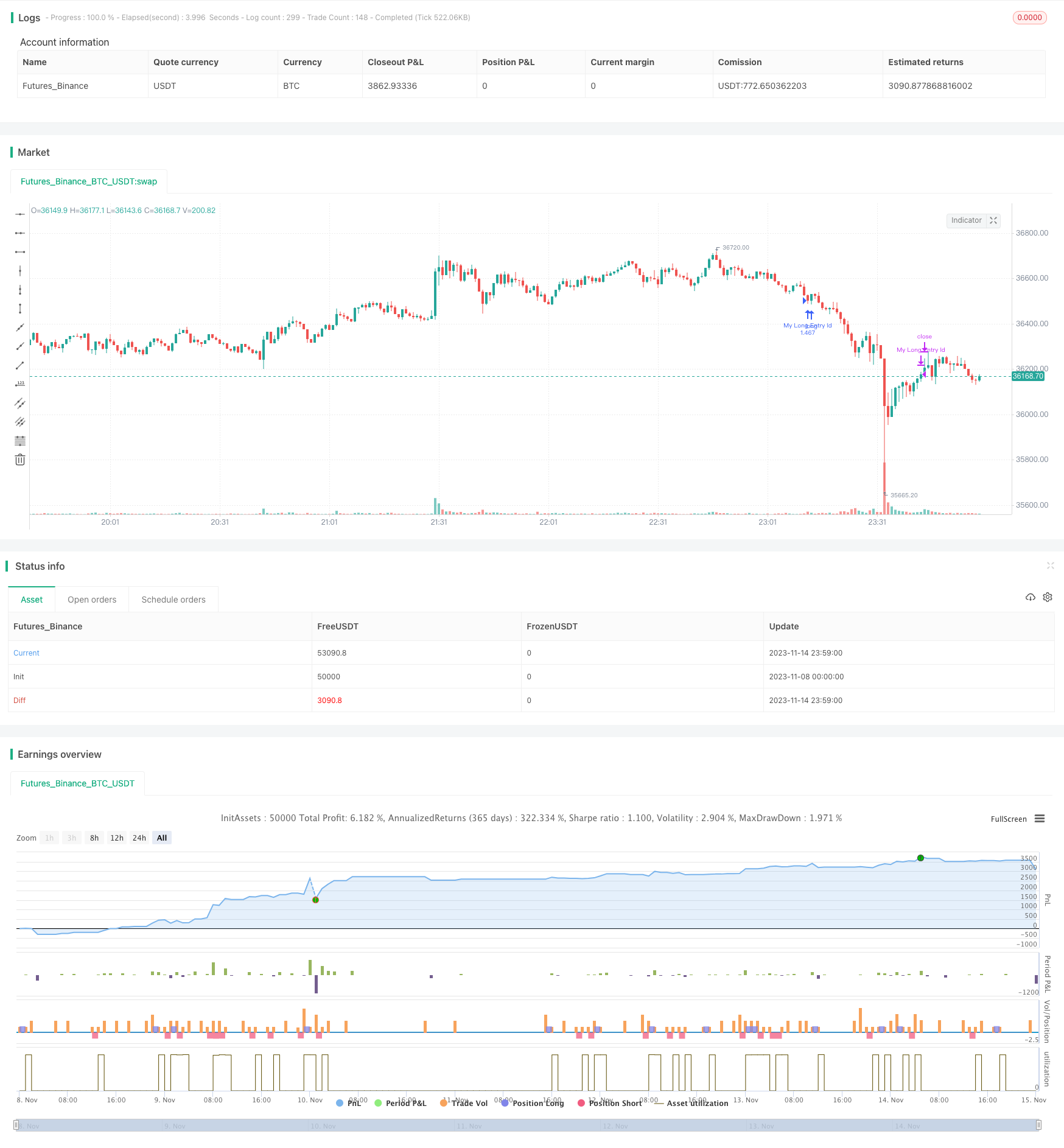# WaveTrend and CMF Based Trend Following Strategy

Author: ChaoZhang, Date: 2023-11-16 16:38:03
Tags:### Overview

This strategy combines WaveTrend indicator and Chaikin Money Flow (CMF) indicator to identify trend direction and follow trends. It runs on 15-minute timeframe, using WaveTrend to determine price trend and CMF to confirm the trend, thus implementing ultra short-term trend following.

### Strategy Logic

WaveTrend indicator can effectively identify the trend direction of prices. It consists of channel midline, channel average and channel index. The channel midline is an exponential moving average of price, reflecting price trend. The channel average is a moving average of channel midline, used to locate channel midline. The channel index reflects the degree of deviation of price from channel midline and generates overbought/oversold signals.

The CMF indicator can judge the inflow and outflow of funds and confirm trends. This indicator is based on the accumulation/distribution line adjusted by volume, reflecting the comparison of buying and selling power. A value around 0 indicates a balance between fund inflow and outflow. Below 0 indicates fund outflow and above 0 indicates fund inflow.

This strategy runs on 15-minute timeframe. It first uses WaveTrend indicator to determine price trend direction, then uses CMF indicator to confirm, so as to follow trends. Specifically, when WaveTrend channel index is below -60 and CMF is less than -0.2, it goes long. When WaveTrend channel index is above 60 and CMF is greater than 0.2, it goes short. The exit conditions are mainly based on CMF indicator - it closes long position when CMF is greater than 0.18, and closes short position when CMF is less than -0.18.

1. WaveTrend indicator can effectively determine price trend direction.
2. CMF indicator can confirm trend direction and avoid wrong trades.
3. Combining WaveTrend and CMF can achieve ultra short-term trend following.
4. 15-minute timeframe makes it more suitable for short-term trading.

### Risk Analysis

1. WaveTrend may generate wrong signals during consolidation.
2. CMF may lag and miss trend turning points.
3. Trading on single timeframe has higher risks, should expand holding period.
4. Lack of stop loss strategy, unable to control single loss.

Solutions:

1. Add other indicators for confirmation to avoid wrong signals.
2. Adjust CMF parameters for higher sensitivity.
3. Expand holding period to lower risks on single timeframe.
4. Add moving stop loss, breakeven stop etc to control loss.

### Optimization

1. Add position sizing for better trend following.
2. Add stop loss strategy to limit single loss.
3. Add indicators like stochastics to avoid errors from single indicator.
4. Test different holding periods to find optimal.
5. Optimize CMF parameters to find best combination.

### Summary

This strategy uses WaveTrend to determine trend and CMF to confirm, for ultra short-term trend following. Its advantages lie in reasonable indicator combination and effective trend following, with 15-minute timeframe making it suitable for short-term trading. But risks exist such as inaccurate signals and overshort holding period. Future improvements like stop loss, parameter optimization and more signal filtering can further enhance its stability and profitability.

```/*backtest
start: 2023-11-08 00:00:00
end: 2023-11-15 00:00:00
period: 1m
basePeriod: 1m
exchanges: [{"eid":"Futures_Binance","currency":"BTC_USDT"}]
*/

//@version=3
strategy(title = "CMF - WaveTrend", shorttitle = "CMF - WaveTrend", overlay = true, pyramiding = 0, default_qty_type = strategy.percent_of_equity, default_qty_value = 100, currency = currency.EUR)

//Chaikin Money Flow

len = input(20, minval=1, title="Length")
mas = input(title="Aggregation", defval="SUM", options=["SUM", "EMA", "WMA"])
e = input(10.0, title="Volume Exponent (0-10 reduces & 10+ increases volume effect)")
p = input(false, title="Show in Percentage")
mvs = input(false, "Factor in Price (Money Volume)")
src=input(hlc3, title="Source for price factor")

trl = min(low,close), trh = max(high,close) // 'true range' fixes issues caused by gaps in price
wv = pow(volume,e/10.0)*(mvs ? src : 1)
ad = (trh==trl ? 0 : (2*close-(trh+trl))/tr(true))*wv
cmf = mas=="SUM" ? sum(ad, len)/sum(wv, len) : mas=="EMA" ? ema(ad, len)/ema(wv, len) : mas=="WMA" ? wma(ad, len)/wma(wv, len) : na
cmf_p  = if p
50*cmf+50
else
cmf
b = p ? 50 : 0

//WaveTrend
n1 = input(10, "Channel Length")
n2 = input(21, "Average Length")
obLevel1 = input(60, "Over Bought Level 1")
obLevel2 = input(53, "Over Bought Level 2")
osLevel1 = input(-60, "Over Sold Level 1")
osLevel2 = input(-53, "Over Sold Level 2")

ap = hlc3
esa = ema(ap, n1)
d = ema(abs(ap - esa), n1)
ci = (ap - esa) / (0.015 * d)
tci = ema(ci, n2)

wt1 = tci
wt2 = sma(wt1,4)
//

longCondition = wt1 < -60 and cmf < - 0.20
if (longCondition)

strategy.entry("My Long Entry Id", strategy.long)

shortCondition = wt1 > 60 and cmf > 0.20
if (shortCondition)

strategy.entry("My Short Entry Id", strategy.short)

closeLongCondition = cmf_p > 0.18 ? true : false
closeShortCondition = cmf_p < -0.18 ? true : false

strategy.close("My Long Entry Id", when=(closeLongCondition == true))
strategy.close("My Short Entry Id", when=(closeShortCondition == true))
```

More